# 第七章-----内外部函数 及 变量

### 1.形参 与 实参

（1）传递给 形参 的 实参 可以是常量、变量或表达式

例如：max(3,a+b);

（2）实参 与 形参的类型应相同或赋值兼容

例如：实参为 int型 而 形参x 为float型，则按不同的类型数值的赋值规则进行转换

### 2.函数调用过程

（1）未被调用时不占用内存，被调用时开系统栈 动态调用内存，调用结束后 形参及函数内存被释放

（2）返回值类型 应与函数类型 相统一，如果 函数值类型 和 return返回类型 不一致，则以函数类型为准

### 3.数组传参

（1）数组名传参传的是 地址，数组元素传参传的是 值

（2）一维数组 传地址：

1.float aver(float *a)
2.float aver(float a[])
3.float aver(float a)

（3）传递地址后，数组中各个元素得一一对应，实参数组与形参数组类型一致，编译时把形参数组名处理为指针变量

，用来接收一个地址

（4）对维数组 传地址：数组是按行存放的，由于形参数组与实参数组类型相同，所以是具有相同长度的一维数组

void Func(int array);
void Func(int array[]);
Func(a);//函数调用  实参直接写数组名！数组名！（重点）

void function(int (*a)[n]);
//不是(int *a[n])(指针数组) ,而是(int (*a)[n])(数组指针);
// 缘由是 [] 的 优先级比 *的大 ，还要注意 * 的从右自左的右结合性
// 即 指针a的类型是右面的参数类型
function(a);//函数调用  实参同样直接写数组名！

void function(int **a,int n);n表示第二维数组长度,即列宽

function( (int **)a,int n);//函数调用//实参不能为数组名！*与上面相反*！有两个 * ！
//(注意)function(a)或function((int *)a)不可以！//因为 int **a 是把二维数组强转为了指向指针的指针
//**a是指向指针的指针，赋值给a的实参必须是指针型变量


#include<stdio.h>
void func(int n, int str[] )
{
int i;
for(i = 0; i < n; i++)
printf("/nstr[%d] = %d/n", i, str[i][i]);
}
int main()
{
char* p;
int str = {{1,2},{3,4},{5,6}};
func(3, str);
}

#include<stdio.h>
void func(int n, int (*str) )
{
int i;
for(i = 0; i < n; i++)
printf("/nstr[%d] = %d/n", i, str[i][i]);
}
int main()
{
char* p;
int str = {{1,2},{3,4},{5,6}};
func(3, str);
}

#include<stdio.h>
void func(int n, int **str )
{
int i;
for(i = 0; i < n; i++)
printf("/nstr[%d] = %d/n", i, *((int*)str + n*i + i));
}
int main()
{
char* p;
int str = {{1,2},{3,4},{5,6}};
func(2, (int**)str);//必须把 二维数组 str的类型强转的和形参类型相同
}
#include <stdio.h>
void func(int n, char **str)
{//**str是指向指针的指针，赋值给str的实参必须是指针型变量
int i;
for(i = 0; i < n; i++)
printf("/nstr[%d] = %s/n", i, str[i]);
}
void main()
{
char* p;//指针数组
char str[] = {"abc","def","ghi"};
p = &str;
p = str;
p = str;
func(3, p);//给**str的其实是 指针数组p的首地址，好在p也是指针变量
//说白了就是数组调用
}

#include <stdio.h>
void func(int n, char  （*str） )
{
int i;
for(i = 0; i < n; i++)
printf("/nstr[%d] = %s/n", i, str[i]);
}

void main()
{
char* p;
char str[] = {"abc","def","ghi"};
func(3, str);
}

#include <stdio.h>
void func(int n, char  str[ ] )
{
int i;
for(i = 0; i < n; i++)
printf("/nstr[%d] = %s/n", i, str[i]);
}

void main()
{
char* p;
char str[] = {"abc","def","ghi"};
func(3, str);
}

### 4.局部变量 与 全局变量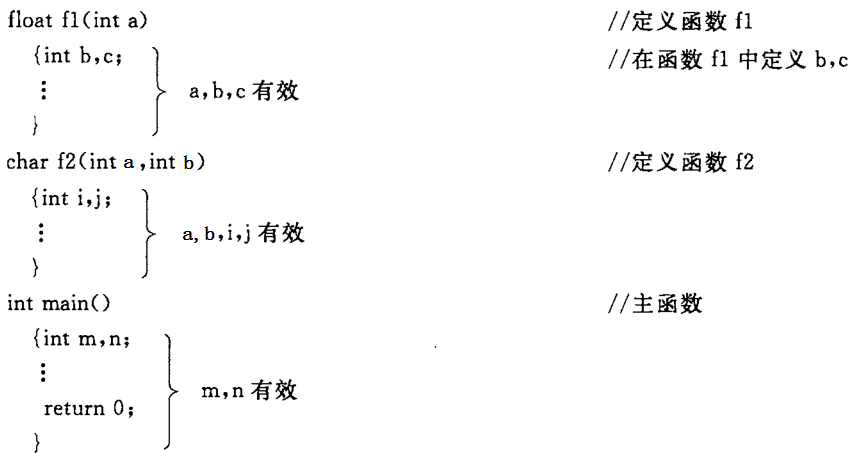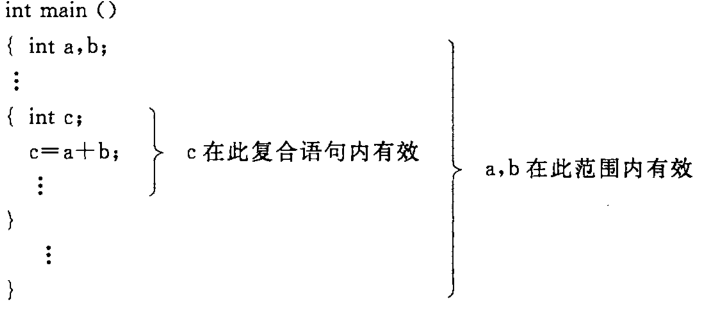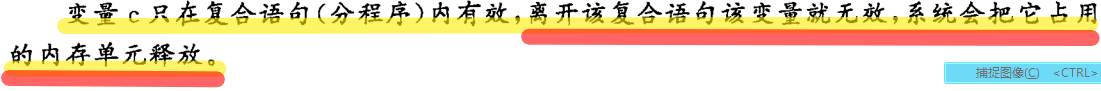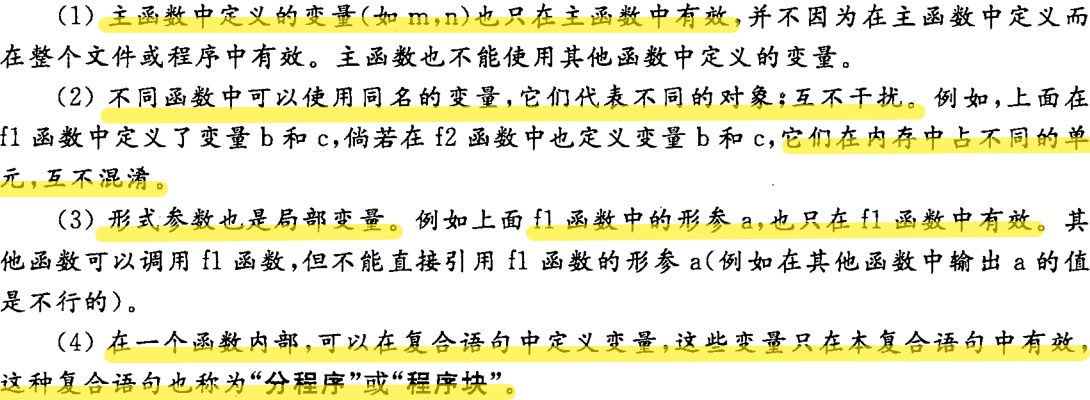（1）在定义此变量的本源文件内：由于编译是从上到下依次编译的,所以函数外定义的全局变量的作用域是定义的位置一直到本文件结束位置。

（2）全局变量,局部变量就跟上下级似得,你上级理论上管的事是多,可还是县官不如现管.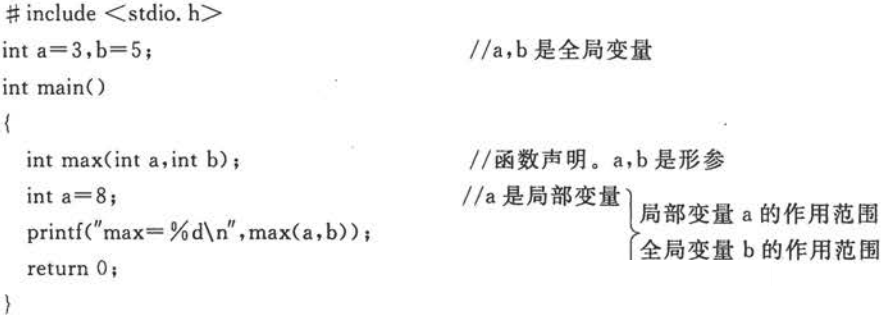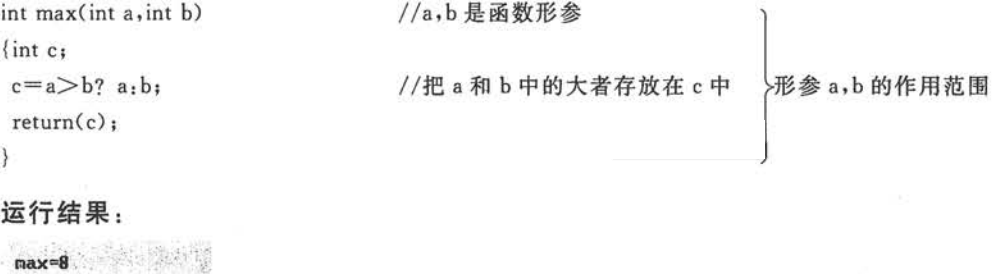### 5.变量的分类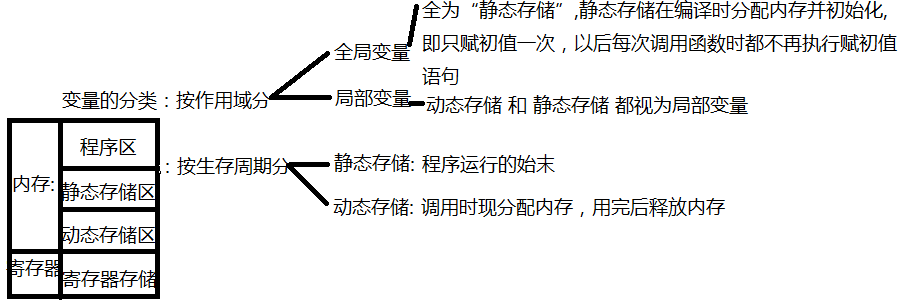### 6.全局变量 作用域的扩展

#include <stdio.h>
int main(){
int max(int,int);
extern int a,b,c;
或者 extern a,b,c；
printf("%d",max(a,max(b,c)));
return 0;
}

int a=1,b=2,c=3;

int max(int a,int b){
return a>b?a:b;
}

例子：在编译连接时，系统知道 NUM 有外部链接，然后 遍历本文件和其他文件 去找外部变量NUM的定义，找到了 NUM 的定义就在调用文件中引用，找不到就报错。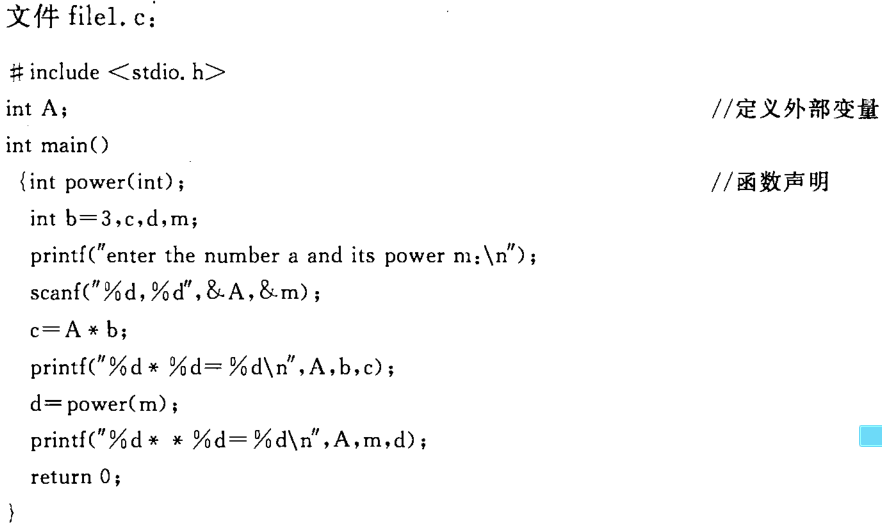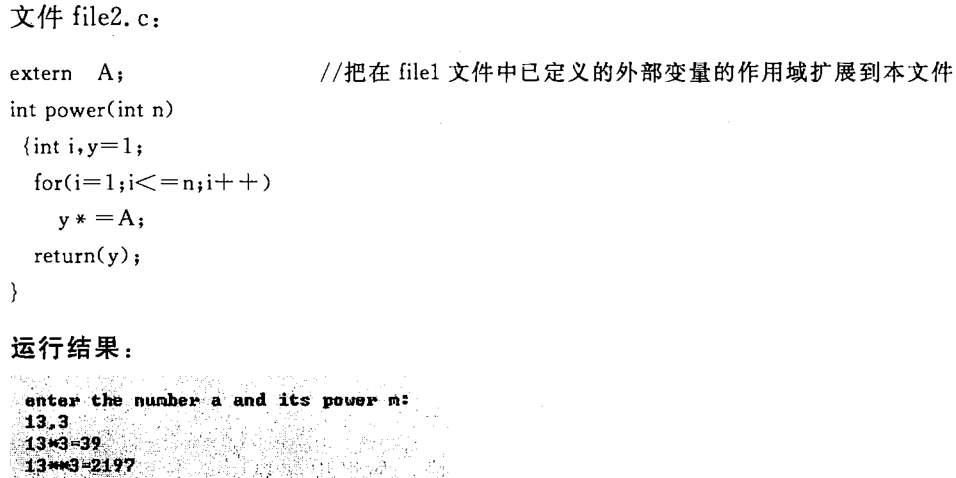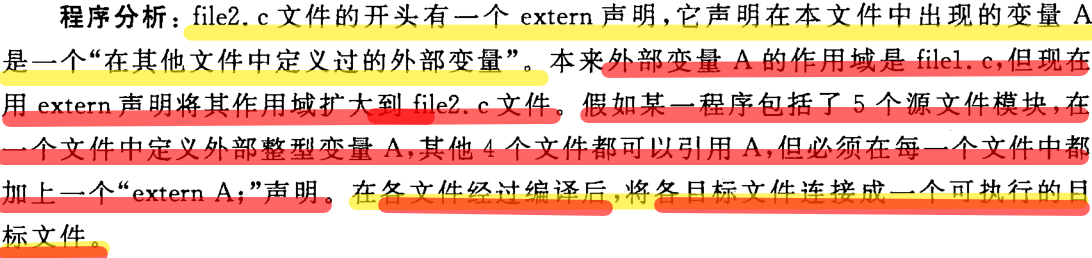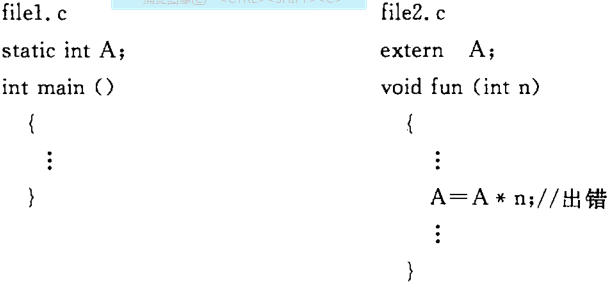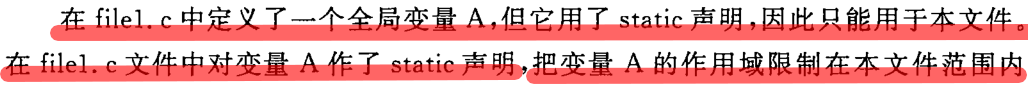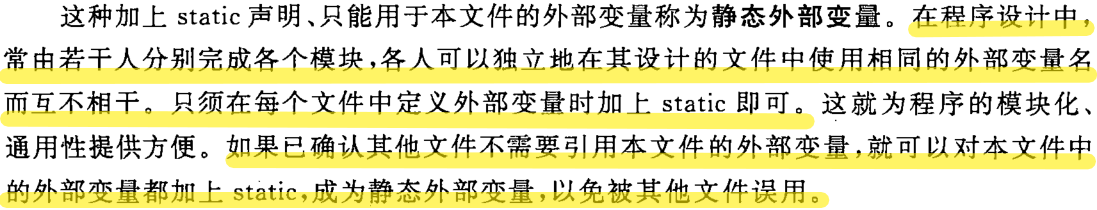### 7.内部函数 外部函数

static int fun(int a,int b) 静态函数声明
extern int fun(int a,int b) 外部函数声明
int fun(int a,int b) 外部函数声明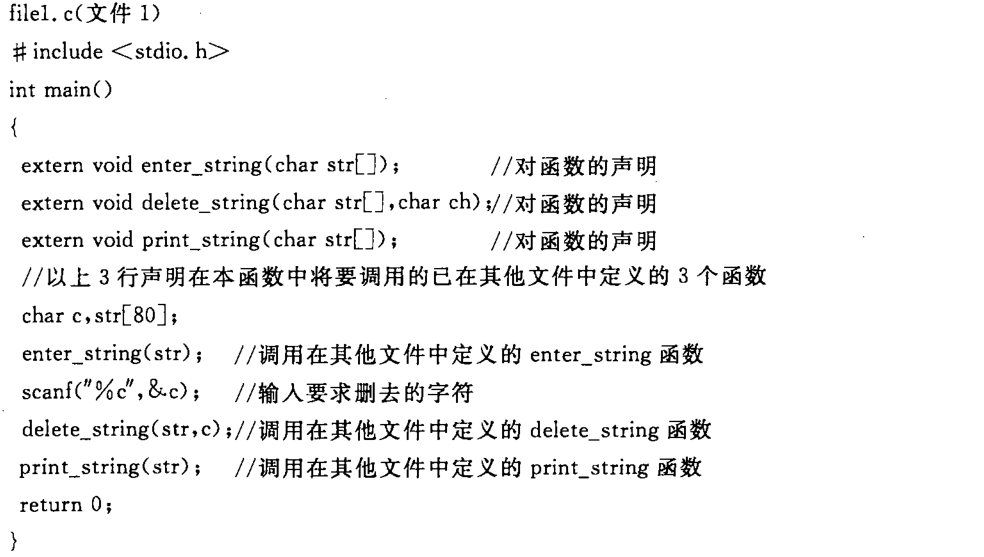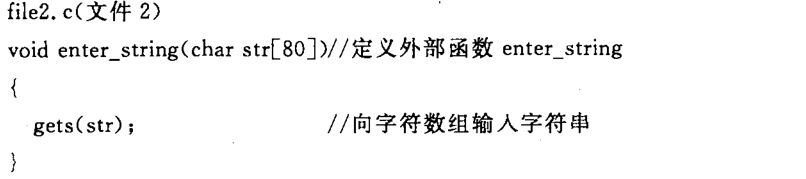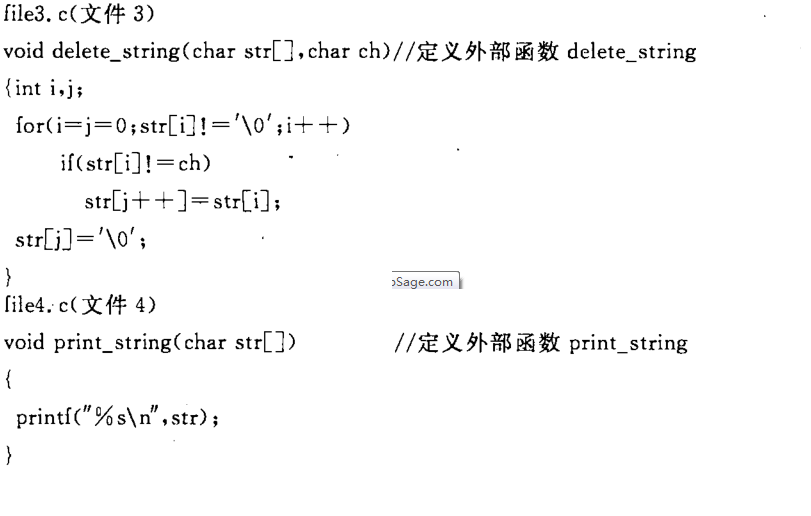double sin(double); //声明给调用函数的文件，标明参数，以便让编译系统判别程序有误与否
#include<math.h>    //调用头文件，将函数的作用域扩展到此文件

©️2019 CSDN 皮肤主题: 技术黑板 设计师: CSDN官方博客